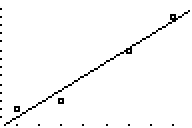# Knowledge Base

## Solution 11918: Calculating and Graphing a Linear Regressions on the TI-83 Plus.

### How do I calculate and graph a linear regression on the TI-83 Plus?

The following example will demonstrate how to calculate a linear regression:

First you will want to enter the data:

1) From the home screen press the [STAT] key to go to the statistics menu.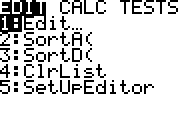2) Next press the  key to go the edit menu.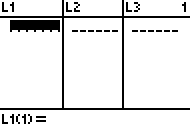3) In the edit menu enter the data {3,5,8,10} in the L1 list and {7,8,14,18} in the L2 list, pressing enter after each entry to move down along the list.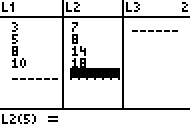Next to calculate the Linear Regression (ax+b):

1) First press the [STAT] key to enter the statistics menu.2) Then press the [right arrow] key to reach the CALC menu and then press the  key to select LinReg(ax+b).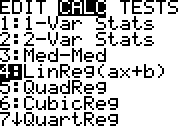3) Next in order input which lists to use for the regression, press the [2nd] key, then the  key to bring up your L1 list, next press the [,] key to separate your two lists and last press the [2nd] key and the  key to bring up your L2 list. Press [,] [VARS] and scroll to the right, now press  .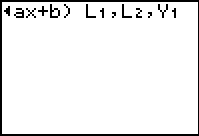4) Press the [ENTER] key to perform the regression calculation.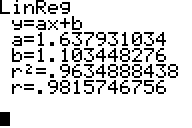The graphing calculator will display the form of the equation as (y=a+bx) and list the values for the two coefficients (a and b). It will store the regression equation to your Y1 function. The correlation coefficient (r and r^2) will be displayed if the diagnostics are on.

Please Note: To turn the diagnostics on, from the home screen, press the [2ND] key and then press the [CATALOG] key. Then in the catalog press the [X-1] key to go down to the letter "D". Last scroll down through the list and select the instruction DiagnosticOn, and press the [ENTER] key twice and it should show DONE.

In order to graph the data:

1) Press then [2nd] key and then press the [STAT PLOT] key to enter the stat plot menu, next press the  key to enter plot 1.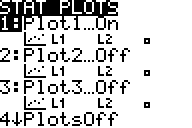2) In the next menu highlight ON and then press the [ENTER] key to turn on the stat plot, and be sure that Xlist is L1 and Ylist is L2.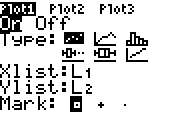3) Next press the [ZOOM] key and then press the  key to set up ZoomStat.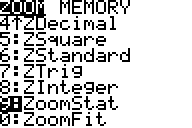4) Your graph screen will display the following: